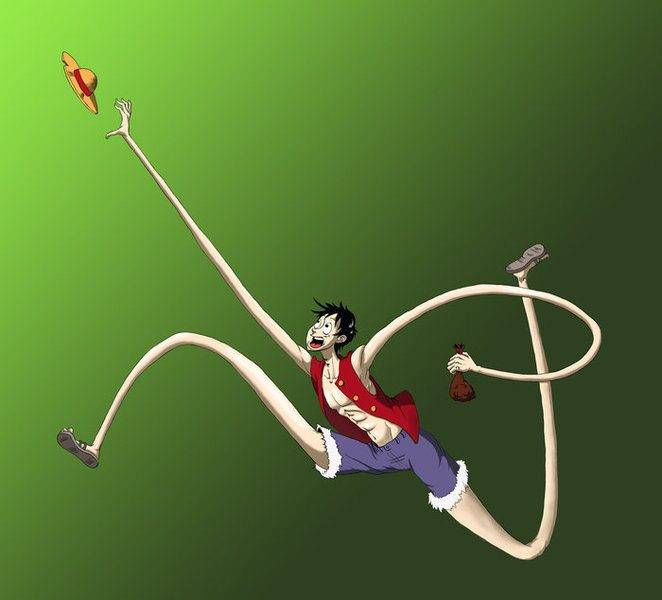# Femur Compression/Young's Modulus

## Homework Statement

By what amount does the 52-cm-long femur of an 73 kg runner compress at this moment? The cross-section area of the bone of the femur can be taken as 5.2×10-4 m2 and its Young's modulus is 1.6×1010 N/m2.

Express your answer to two significant figures and include the appropriate units.

## Homework Equations

Stress on femur = F/A
Strain = delta L/L = (1/Y)(F/A)

## The Attempt at a Solution

F/A = (73 kg * 9.8 m/ss)/(5.2 * 10-4 m2) = 1375769.231 N/m2

deltaL/L = (1/1.6×1010 N/m2)(1375769.231 N/m2) = 8.598557692 * 10-5

(8.598557692 * 10-5) * 0.52 m = 4.47125 * 10-5 m = 4.5 * 10-5 m

I'm not sure what I'm doing wrong; any help would be appreciated!

Last edited:

SteamKing
Staff Emeritus
Homework Helper

## Homework Statement

By what amount does the 52-cm-long femur of an 73 kg runner compress at this moment? The cross-section area of the bone of the femur can be taken as 5.2×10-4 m2 and its Young's modulus is 1.6×1010 N/m2.

Express your answer to two significant figures and include the appropriate units.

## Homework Equations

Stress on femur = F/A
Strain = delta L/L = (1/Y)(F/A)

## The Attempt at a Solution

F/A = (73 kg * 9.8 m/ss)/(5.2 * 10-4 m2) = 1375769.231 N/m2

deltaL/L = (1/1.6×1010 N/m2)(1375769.231 N/m2) = 8.598557692 * 10-5

(8.598557692 * 10-5) * 0.52 m = 4.47125 * 10-5 m = 4.5 * 10-5 m

I'm not sure what I'm doing wrong; any help would be appreciated!
What makes you think you're doing anything wrong?

BTW, the femur is one of the biggest and strongest bones in the human body. It's not supposed to shrink or stretch very much under normal use.

Unless you're this guy:•billy_joule
This was a problem on MasteringPhysics and when I submitted this answer, it was incorrect. :(

SteamKing
Staff Emeritus
Homework Helper
This was a problem on MasteringPhysics and when I submitted this answer, it was incorrect. :(
Your problem statement seems to start in the middle. It's asking you to calculate by what amount the femur compresses "in this moment", but it is not clear what that means. Your calculation assumes that all the static weight of the runner is supported by one femur. Is that what the problem is really asking you? When someone is running, dynamic loadings on the legs can easily exceed the loads present when one is standing still.

There was also this bit of information in the problem that I assumed wasn't important:

"The normal force of the ground on the foot can reach three times a runner's body weight when the foot strikes the pavement."

Should I be multiplying the force by 3?

SteamKing
Staff Emeritus